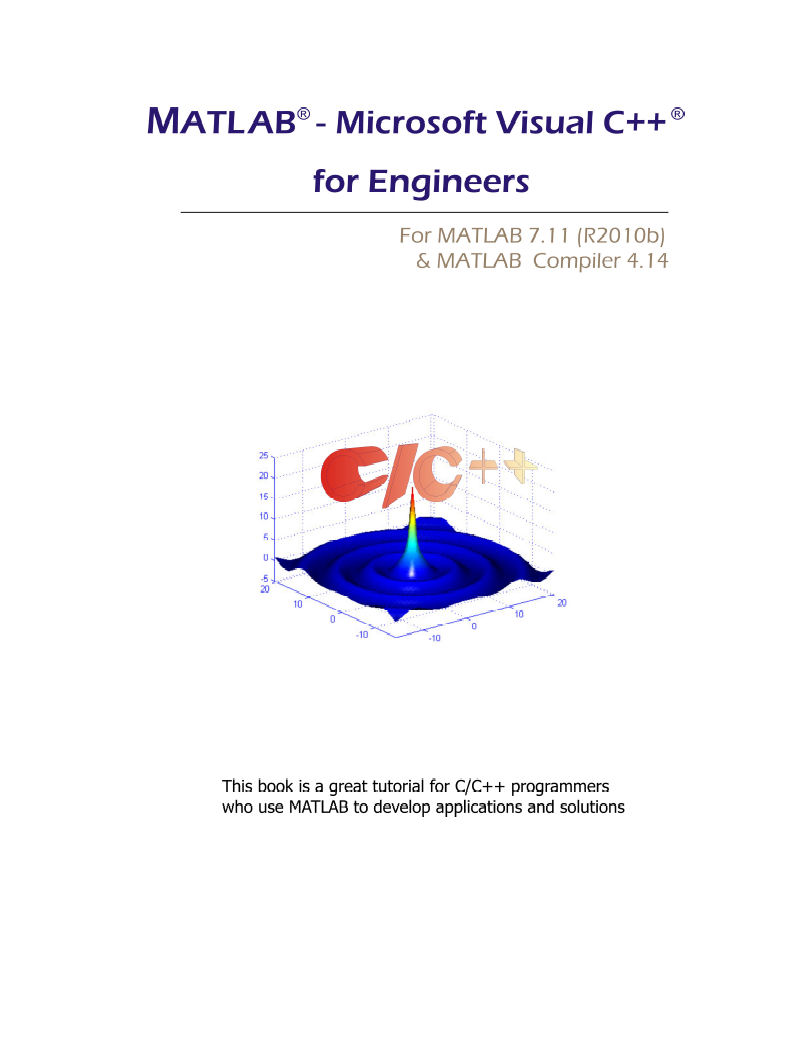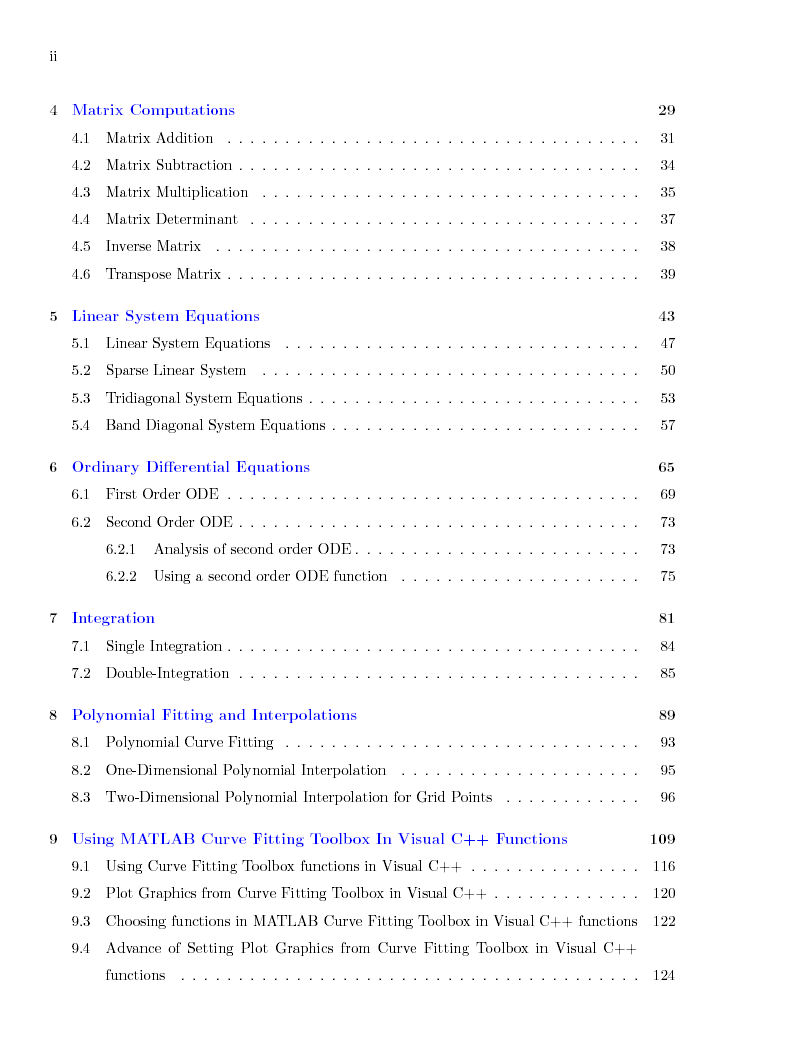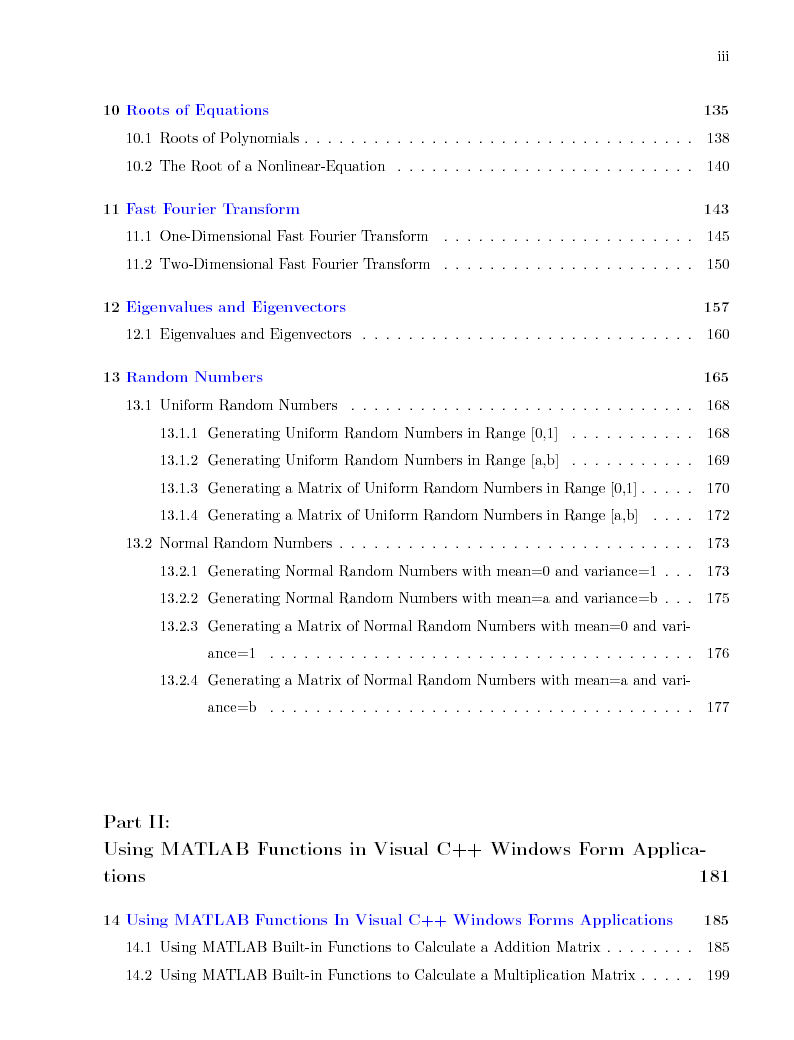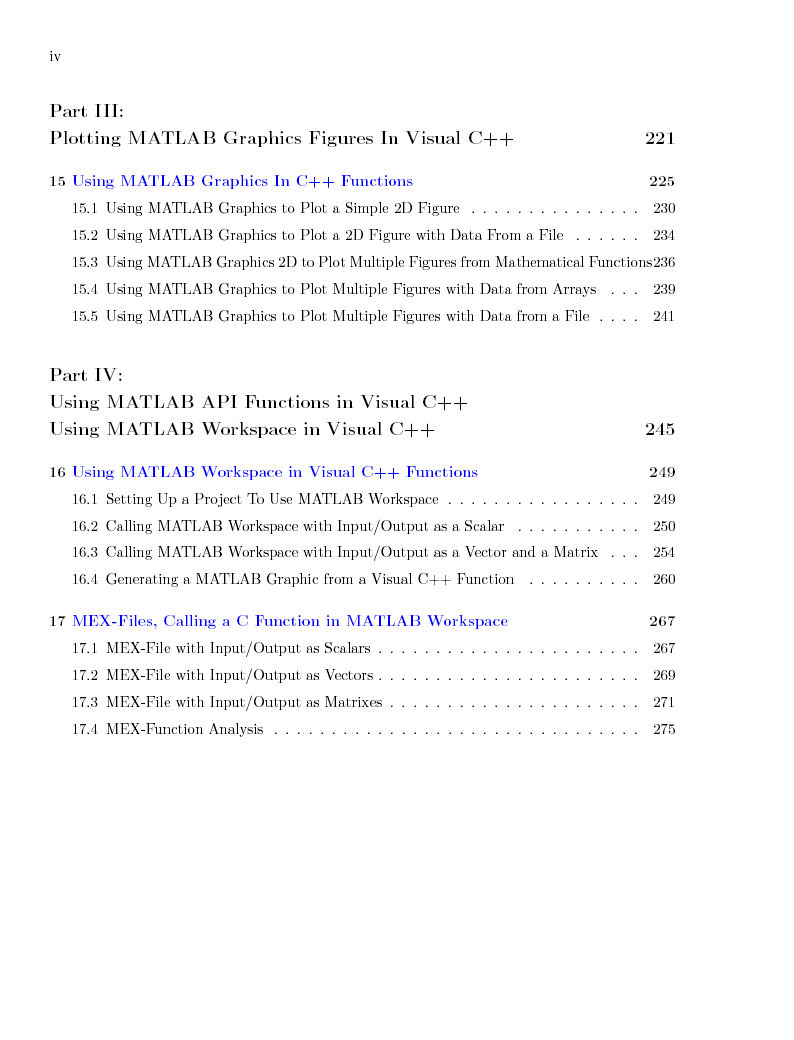MATLAB® - Microsoft Visual C++®   for Engineers (ebook)

For MATLAB 2010(b) and MATLAB Compiler 4.14

MATLAB - Microsoft Visual C++ for Engineers book illustrates the works between MSVC++ programming and MATLAB. The  book describes very detail how to use MATLAB built-in functions in VB applications. If you are a MSVC++ programmer and want to use the quality mathematical built-in functions in your MSVC++  applications, to solve mathematical problems in MSVC++ programming code, then this book is what you're looking for.

The features of this book are designed to handle following topics:

1. MSVC++ functions call MATLAB built-in functions in the class created from MATLAB M-files to solve the mathematical problems.

2. MSVC++Windows Applications uses MATLAB built-in functions.

3. MSVC++ functions plot figures from MATLAB Graphics.

4. MSVC++ functions use API functions.

5. MSVC++ functions use MATLAB Curve Fitting Toolbox functions.

This book is an ebook in the pdf format and MSVC++ projects which includes the complete MSVC++ code examples of all chapters . The example codes are developed, compiled, and tested in  MATLAB 2010(b) and MATLAB Compiler 4.14 with Microsoft Visual Studio 2008 on Windows 7. The examples are working on scalars, vectors, and matrixes which are inputs/outputs of functions for every application. In addition, the example codes are portable and presented in the step-by-step method, therefore you can easily reuse the codes or write your own codes following the step-by-step procedure.

This book is a great support for MSVC++ programmers who are using MATLAB built-in functions to develop applications and solutions. Using the combination of both tools, MSVC++  and MATLAB, you have the best tool in your hand to develop and solve your  technical problems.

MATLAB - Microsoft Visual C++ for Engineers (ebook)

MATLAB and MATLAB Compiler are registered trademarks of The MathWork, Inc.
Microsoft, Windows, and Microsoft Visual Basic .NET areMATLAB - Visual Basic .NET for Engineers